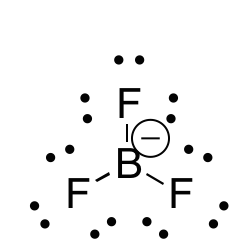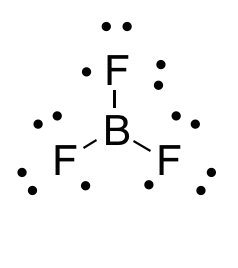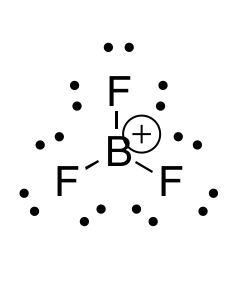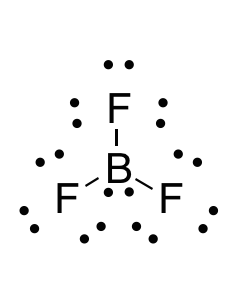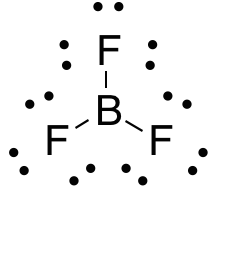# High School Chemistry : Compounds and Bonding

## Example Questions

### Example Question #2 : Help With Covalent Bonds

Which of the following describes a covalent bond?

An electron is donated from one atom to another

A proton is donated from one atom to another

A proton is shared between two atoms

An electron is shared between two atoms

Two or more electrons are shared between two atoms

Two or more electrons are shared between two atoms

Explanation:

There are two primary types of intramolecular bonds: ionic bonds and covalent bonds. In an ionic bond, an electron is transferred (donated) from one atom to the other, usually allowing both atoms to satisfy the octet rule. In a covalent bond, electrons are shared between two atoms in order to allow both to satisfy the octet rule. A covalent single bond consists of two shared electrons, while a double bond will consist of four and a triple bond will consist of six.

Covalent bonds usually form between two non-metals. Diatomic gases, CH4, and NaOH are all examples of molecules that contain covalent bonds.

### Example Question #81 : Elements And Compounds

What is the term for a covalent bond in which electrons are not shared equally?

Non-polar covalent bond

Coordinate covalent bond

Ionic bond

Polar covalent bond

Double bond

Polar covalent bond

Explanation:

A polar covalent bond describes a bond between two atoms that share electrons unequally. This results from a difference in electronegativity between the atoms. This difference is enough to cause a dipole, but not enough to consitute an ionic bond.

A non-polar covalent bond is a bond between two atoms sharing ions equally due to similar electronegativities.

A double bond occurs when four electrons are shared between two atoms.

A coordinate covalent bond refers to a covalent bond in which one atom donates both shared electrons.

### Example Question #172 : High School Chemistry

Pauling electronegativity values: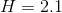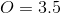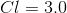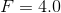Based on the given electronegativities, which of these bonds would most likely be a nonpolar covalent bond?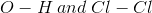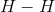only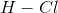only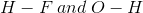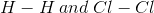Explanation:

A nonpolar covalent bond occurs when two atoms share electrons equally. This happens when the electronegativities of each atom is relatively close to one another. For example, in water, oxygen is much more electronegative than hydrogen (3.5 and 2.1 respectively), oxygen keeps the electrons closer to its nucleus and results in uneven sharing of the electrons between itself and the two hydrogen atoms. This results in a net dipole in the molecule with the oxygen-end being slightly negative, and the hydrogen-end being slightly positive.

### Example Question #1 : Help With Lewis Diagrams

Which of the following compounds breaks the octet rule?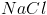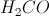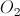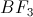Explanation:

The octet rule requires that each atom in the molecule has eight valence electrons, fully filling the s and p subshells. Atoms that have a full octet tend to be more stable and lower in energy.

In the compound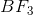, each fluorine has seven initial valence electrons and boron has three initial valence electrons. Upon forming the compound bonds, boron shares its three electrons with each fluorine through covalent bonds. This givens each fluorine a full octet, but leave boron with only three covalent bonds, resulting in only six valence electrons. Boron does not satisfy the octet rule in this compound.

Each atom in the other listed compounds forms bonds to generate a full octet. Sodium chloride achieves this through an ionic bond. Methanal and diatomic oxygen both use double bonds to help achieve octets.

### Example Question #2 : Help With Lewis Diagrams

How many lone pairs of electrons exist on the following molecule?

BF3

11

1

9

10

0

9

Explanation:

..            ..

:F — B —  F:         Boron can have a violated octet (6 e-) and each F has 3 lone pairs

..      |      ..          for a total of 9 pairs of unpaired electrons

:F:

..

### Example Question #2 : Help With Lewis Diagrams

Which of the following compounds contains an atom that does NOT satisfy the octet rule?

I.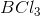II.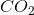III.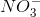IV.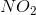I, II, and IV

I and II

II and III

I and IV

IV only

I and IV

Explanation:

BCl3 only has six electrons around boron, while NO2 (with an odd number of electrons) would have only 7 electrons around the central nitrogen.

### Example Question #112 : Compounds And Molecules

Which of the following elements will have two unpaired valence electrons?

Carbon

Fluorine

Neon

Beryllium

Nitrogen

Carbon

Explanation:

Lewis dot diagrams can help us keep track on how the valence electrons will disperse themselves among orbitals in the atom. When drawing these diagrams, it is important to keep two things in mind.

1. The s orbital will be filled before the p orbital begins to be filled.

2. Electrons will never pair in the same orbital if an orbital of equal energy is unoccupied.

Knowing these two facts, we can predict which of the following elements will have two unpaired electrons.

Fluorine will have seven valence electrons, meaning that only one orbital will not be completely filled.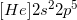Beryllium only has two valence electrons, but they will both be found in the 2s orbital, because it must be filled before the p orbitals can receive electrons.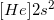Nitrogen will have five valence electrons, so two will be found in the 2s orbital. The other three will be present in their own p orbitals, meaning nitrogen has three unpaired electrons.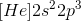Neon will have eight valence electrons, fully filling the 2s and 2p orbitals. It will have no unpaires electrons.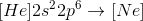Carbon has one less valence electron than nitrogen, meaning that it will have two unpaired electrons in the p orbitals.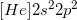### Example Question #2 : Lewis Diagrams

Which of the following is isoelectric with the cation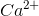?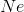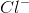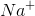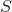Explanation:

Isoelectronic means having the same electron configuration (i.e. the same number of electrons).andboth have the same number of electrons (18), while sulfur has 16, phosphorous has 15, neon has 10, and the sodium cation has 10.

### Example Question #1 : Help With Lewis Diagrams

Which answer option correctly depicts the Lewis dot structure of sodium chloride?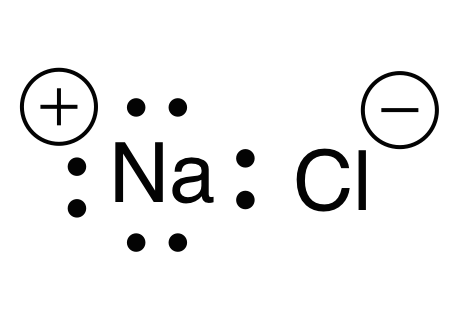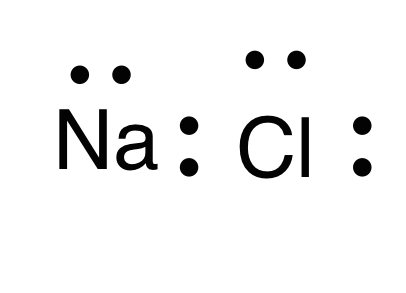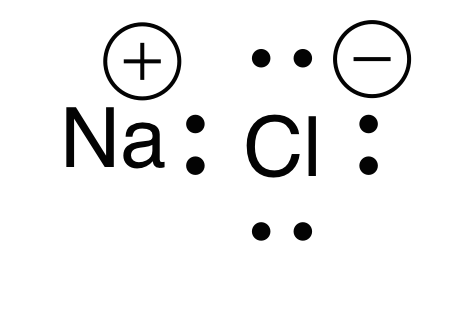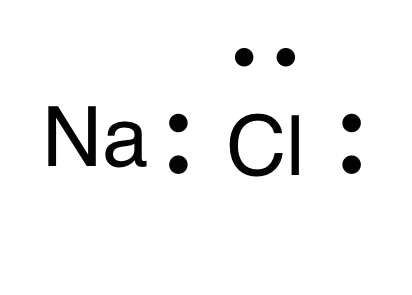Explanation:

When drawing a Lewis dot structure, we are always trying to reach an electron count where all atoms involved are stable and (usually) have full octets. We are also trying to estabilsh a structure in which we have the smallest formal charge possible. The general rule is first to draw out all of the elements involved and their valence electrons, then start piecing them together trying to reduce the formal charge and get all elements involved to an octet. There are a couple exceptions to the octet rule.

Sodium and chlorine form an ionic bond, meaning that one atom will donate an electron and the other will receive it. This gives each atom a charge. Chlorine has seven valence electrons, while sodium has one valence electron. For each atom to arrive at an octet, sodium will need to lose one electron and chlorine will need to gain one electron. This would give chlorine a negative charge, and sodium a positive charge.

Thus, the answer is a sodium with a positive charge (due to one lost electron) and a chlorine with eight electrons and a negative charge (due to one electron gained).

### Example Question #1 : Diagrams And Geometry

What is the Lewis dot structure for?